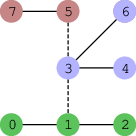Your browser (Unknown 0) is no longer supported. Some parts of the website may not work correctly. Please update your browser.

#### TreeProduct

Remove at most two edges from a tree graph to maximize the product of the components' sizes.

Elves in the forest of Glandishar are preparing for an Orc invasion. They have a network of N + 1 guard posts located on the open platforms in the treetops. The posts are numbered from 0 to N and are connected by N bridges, so that one can get from any one guard post to any other guard post in a unique way. In other words, guard posts and bridges form a tree graph.

The Elves are afraid that if the Orcs manage to get hold of one of the guard posts, then they will have easy access to all the other guard posts. Therefore, the Elves have decided to destroy at most two bridges and split the guard posts into at most three separate areas, so that the guards can move within each area but it's not possible to move between the areas.

In each area there will be one guard who will move from guard post to guard post during the night. If the Orcs attack, the guards will raise an alarm. However, if the Orcs manage to guess the guard post in which the guards are currently located, they may manage to take out the guards without raising the alarm. The Elves want to avoid the situation that all guard posts fail this way, by maximizing:

• X * Y * Z, if the guard posts have been divided into three areas consisting of X, Y and Z guard posts, respectively;
• X * Y, if the guard posts have been divided into two areas consisting of X and Y posts, respectively;
• N + 1, if the guard posts haven't been divided;

depending on which option gives the largest result.

You are given a map of the network in the form of two arrays A, B of length N. For each K (0 ≤ K < N) there is a bridge between posts A[K] and B[K].

Write a function:

class Solution { public String solution(int[] A, int[] B); }

that, given two arrays A and B of N integers, returns the largest possible result. Since the result can be large, you should return it as a string.

For example, given the following arrays:

A = 0 B = 1 A = 1 B = 2 A = 1 B = 3 A = 3 B = 4 A = 3 B = 5 A = 6 B = 3 A = 7 B = 5the function should return "18" since the Elves can destroy bridges 1−3 and 3−5 (marked as dashed lines in the image above). The created areas consist of 3, 3 and 2 guard posts.

Therefore, the result is 3 * 3 * 2 = 18. It is not possible to obtain a better result.

Given the following arrays:

A = 0 B = 1 A = 1 B = 2

the function should return "3" (it is optimal not to destroy any bridge).

Write an efficient algorithm for the following assumptions:

• N is an integer within the range [1..50,000];
• each element of arrays A, B is an integer within the range [0..N];
• distance from guard post 0 to any other post is not greater than 900 bridges.